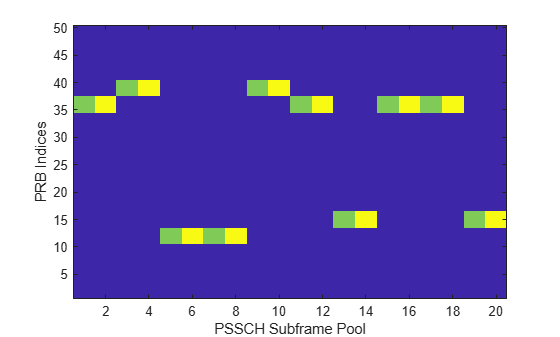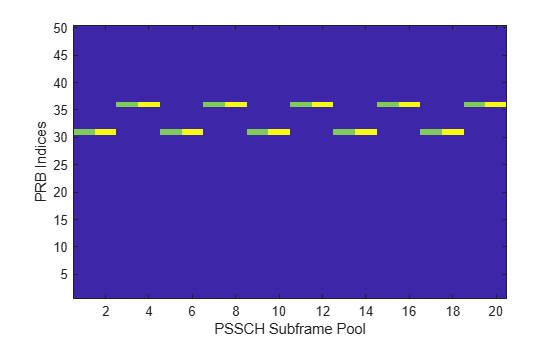# lteSCIResourceAllocation

SCI message physical resource blocks allocation

## Syntax

``prbset = lteSCIResourceAllocation(ue,scistr)``

## Description

example

````prbset = lteSCIResourceAllocation(ue,scistr)` returns a column vector containing the zero-based physical resource block (PRB) indices for the specified UE settings and as defined by the resource allocation substructure of the sidelink control information (SCI) message structure. The PRB indices created are for a single PSSCH transmission in a subframe within the PSSCH subframe pool. For more information, see SCI Resource Allocation.```

## Examples

collapse all

Display the PRB allocations associated with the sequence of subframes in a PSSCH subframe pool.

Configure a nonhopping allocation of 3 PRBs according to the RIV calculation specified in TS 36.213, Section 8.1.1.

```ue = struct('NSLRB',50); sci = struct('FreqHopping',0); sci.Allocation.RIV = 110;```

Display an image of the PRBs used in each slot of each subframe in a pool of 10 PSSCH subframes.

```subframeslots = zeros(ue.NSLRB,20); for i = 0:9 ue.NSubframePSSCH = i; prbSet = lteSCIResourceAllocation(ue,sci); prbSet = repmat(prbSet,1,2/size(prbSet,2)); for s = 1:2 subframeslots(prbSet(:,s)+1,2*i+s) = 20+s*20; end end imagesc(subframeslots) axis xy xlabel('PSSCH Subframe Pool') ylabel('PRB Indices')```Configure a type 2 hopping allocation of 3 PRBs. Display the PRB allocations that are associated with the sequence of subframes in a PSSCH subframe pool.

Configure UE and SCI settings structures for a type 2 hopping allocation of 3 PRBs.

```ue = struct('NSLRB',50); ue.PSSCHHoppingParameter = 10; ue.NSubbands = 2; ue.PSSCHHoppingOffset = 1; sci = struct('FreqHopping',1); sci.Allocation.RIV = 110; sci.Allocation.HoppingBits = 3;```

Display an image of the PRBs used in each slot of each subframe in a pool of 10 PSSCH subframes.

```subframeslots = zeros(ue.NSLRB,20); for i = 0:9 ue.NSubframePSSCH = i; prbSet = lteSCIResourceAllocation(ue,sci); prbSet = repmat(prbSet,1,2/size(prbSet,2)); for s = 1:2 subframeslots(prbSet(:,s)+1,2*i+s) = 20+s*20; end end imagesc(subframeslots) axis xy xlabel('PSSCH Subframe Pool') ylabel('PRB Indices')```Configure a type 1 hopping allocation of 3 PRBs. Display the PRB allocations that are associated with the sequence of subframes in a PSSCH subframe pool.

Configure UE and SCI settings structures for a type 1 hopping allocation of 3 PRBs.

```ue = struct('NSLRB',50); sci = struct('FreqHopping',1); sci.Allocation.RIV = 110; sci.Allocation.HoppingBits = 1;```

Display an image of the PRBs used in each slot of each subframe in a pool of 10 PSSCH subframes.

```subframeslots = zeros(ue.NSLRB,20); for i = 0:9 ue.NSubframePSSCH = i; prbSet = lteSCIResourceAllocation(ue,sci); prbSet = repmat(prbSet,1,2/size(prbSet,2)); for s = 1:2 subframeslots(prbSet(:,s)+1,2*i+s) = 20+s*20; end end imagesc(subframeslots) axis xy xlabel('PSSCH Subframe Pool') ylabel('PRB Indices')```Configure PRB pool restriction for transmission mode 2. Display the PRB allocations that are associated with the sequence of subframes in a PSSCH subframe pool.

Configure a UE settings structure with specified PRB indices. Default settings are used for other UE and SCI fields.

```ue = struct('NSLRB',50); ue.PRBPool = (30:49); sci = struct('FreqHopping',1);```

Display an image of the PRBs used in each slot of each subframe in a pool of 10 PSSCH subframes.

```subframeslots = zeros(ue.NSLRB,20); for i = 0:9 ue.NSubframePSSCH = i; prbSet = lteSCIResourceAllocation(ue,sci); prbSet = repmat(prbSet,1,2/size(prbSet,2)); for s = 1:2 subframeslots(prbSet(:,s)+1,2*i+s) = 20+s*20; end end imagesc(subframeslots) axis xy xlabel('PSSCH Subframe Pool') ylabel('PRB Indices')```## Input Arguments

collapse all

User equipment settings, specified as a parameter structure containing these fields:

Number of sidelink resource blocks, specified as an integer scalar from 6 to 110.

Example: `6`, which corresponds to a channel bandwidth of 1.4 MHz.

Data Types: `double`

PSSCH subframe number in PSSCH subframe pool, specified as an integer scalar. (${n}_{\text{ssf}}^{\text{PSSCH}}$)

Note

This parameter is required for SCI format 0 and frequency hopping. (`scistr`.`SCIFormat` = `'Format0'` and `scistr`.`FreqHopping` = 1)

Data Types: `double`

PSSCH hopping parameter, specified as an integer scalar from 0 to 510. (SL-HoppingConfigComm-r12 {hoppingParameter-r12})

All values ≥ 504 are treated as 510.

Note

This parameter is required for SCI format 0 and frequency hopping. (`scistr`.`SCIFormat` = `'Format0'` and `scistr`.`FreqHopping` = 1)

Data Types: `double`

Number of subbands, specified as 1, 2, or 4. (SL-HoppingConfigComm-r12 {numSubbands-r12})

Note

This parameter is required for SCI format 0 and frequency hopping. (`scistr`.`SCIFormat` = `'Format0'` and `scistr`.`FreqHopping` = 1)

Data Types: `double`

PSSCH hopping offset, specified as an integer scalar from 0 to 110. (SL-HoppingConfigComm-r12 {rb-Offset-r12})

Note

This parameter is required for SCI format 0 and frequency hopping. (`scistr`.`SCIFormat` = `'Format0'` and `scistr`.`FreqHopping` = 1)

Data Types: `double`

PSSCH resource block pool (sidelink transmission mode 2), specified as a zero-based integer vector of indices giving the PRBs in the pool. If `PRBPool` is absent or empty, the pool is assumed to be the full transmission bandwidth.

Note

This parameter is required for SCI format 0 and frequency hopping. (`scistr`.`SCIFormat` = `'Format0'` and `scistr`.`FreqHopping` = 1)

Data Types: `double`

Number of sub-channels in the V2X PSSCH resource pool, specified as an integer scalar from 1 to 110.

Note

This parameter is required for SCI format 1. (`scistr`.`SCIFormat` = `'Format1'`)

Data Types: `double`

Number of PRB in each sub-channel, specified as an integer scalar from 1 to 110.

Note

This parameter is required for SCI format 1. (`scistr`.`SCIFormat` = `'Format1'`)

Data Types: `double`

First PRB index associated with first sub-channel of the resource pool, specified as an integer scalar from 1 to 109.

Note

This parameter is required for SCI format 1. (`scistr`.`SCIFormat` = `'Format1'`)

Data Types: `double`

Whether PSCCH and PSSCH are transmitted in adjacent PRB, specified as `'On'` or `'Off'`.

Note

This parameter is required for SCI format 1. (`scistr`.`SCIFormat` = `'Format1'`)

Data Types: `double`

First sub-channel index of PSSCH resource allocation, specified as an integer scalar from 1 to 109.

Note

This parameter is required for SCI format 1. (`scistr`.`SCIFormat` = `'Format1'`)

Data Types: `double`

Data Types: `struct`

Sidelink control information settings, specified as a parameter structure containing these PRB allocation fields:

SCI format type, specified as `'Format0'` or `'Format1'`.

Data Types: `char` | `string`

Frequency hopping flag, specified as 0 for nonhopping allocation type or 1 for hopping allocation type. When `scistr`.`FreqHopping` = 1, the hopping allocation type is signalled by `scistr`.`Allocation`.`HoppingBits`.

Note

This parameter is required for SCI format 0. (`scistr`.`SCIFormat` = `'Format0'`)

Data Types: `double`

Resource allocation parameter substructure, specified as a structure.

Hopping bits, specified as a bit vector with 0, 1, or 2 bits. The `HoppingBits` parameter signals the hopping type. For more information, see SCI Resource Allocation.

Note

This parameter is required for SCI format 0. (`scistr`.`SCIFormat` = `'Format0'`)

Data Types: `double`

Resource indication value, specified as a bit vector with 5 to 13 bits. The resource indication value assignment for sidelink follows the specifications for uplink, as modified in TS 36.213 , Sections 14.1.1.2 and 14.1.1.4. For more information, see SCI Resource Allocation.

Note

This parameter is required for SCI format 0. (`scistr`.`SCIFormat` = `'Format0'`)

Data Types: `double`

Data Types: `struct`

Resource indication value, specified as a bit vector with 0 to 13 bits. The resource indication value assignment for sidelink follows the specifications for uplink, as modified in TS 36.213 , Sections 14.1.1.2 and 14.1.1.4. For more information, see SCI Resource Allocation.

Note

This parameter is required for SCI format 1. (`scistr`.`SCIFormat` = `'Format1'`)

Data Types: `double`

Data Types: `struct`

## Output Arguments

collapse all

Physical resource block indices, returned as a nonnegative integer column vector or N-by-2 integer matrix of zero-based indices.

• When the allocation type defines one set of PRB indices to use in the first and second slots of the subframe, `prbset` is returned as an integer column vector.

• When the allocation type defines a different set of PRB indices in the first and second slots of the subframe, `prbset` is returned as two-column integer matrix.

The PRB indices created are for a single PSSCH transmission in a subframe within the PSSCH subframe pool.

collapse all

### SCI Resource Allocation

Sidelink control information (SCI) resource allocation mapping is described in TS 36.211 , Section 9.3.6. The `sciout` structure returned by `lteSCI` can be directly used as the `scistr` structure input to `lteSCIResourceAllocation`. Using `lteSCI` creates a properly formatted SCI format 0 message, ensuring that the field values adhere to the underlying field bit lengths. The `scistr` field values are read modulo to the SCI message bit lengths. Any fields missing from `scistr` default to 0. PSSCH allocations are based on uplink resource allocation type 0 (see `lteDCI`, DCI format 0). In these allocations, the same single contiguous PRB allocation must be used for both slots in the subframe. As with uplink, for sidelink:

• A `FreqHopping` value of 1 signals a hopping allocation type. There are two types of hopping: type 1 PUSCH hopping and type 2 PUSCH hopping (frequency hopping with a predefined pattern). `scistr`.`Allocation`.`HoppingBits` signals the hopping type, as specified in TS 36.213 , Table 8.4-2.

• A `FreqHopping` value of 0 signals a nonhopping allocation type

Alternatively, you can use `lteDCIResourceAllocation` with a DCI format 5 message and the same message fields to generate the PSSCH allocations. This PSSCH allocation represents sidelink transmission mode 1, with the eNodeB using a DCI format 5 message to provide the transmitting UE with a PSSCH resource allocation.

 3GPP TS 36.211. “Evolved Universal Terrestrial Radio Access (E-UTRA); Physical Channels and Modulation.” 3rd Generation Partnership Project; Technical Specification Group Radio Access Network. URL: https://www.3gpp.org.

 3GPP TS 36.213. “Evolved Universal Terrestrial Radio Access (E-UTRA); Physical layer procedures.” 3rd Generation Partnership Project; Technical Specification Group Radio Access Network. URL: https://www.3gpp.org.